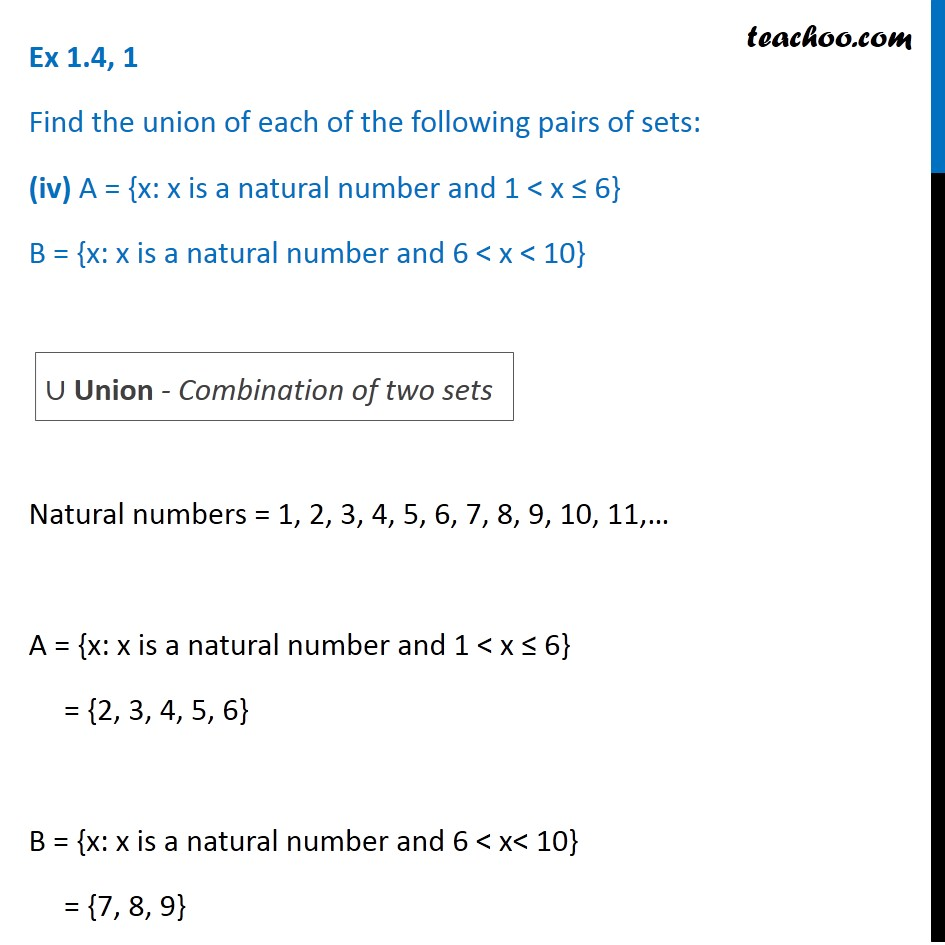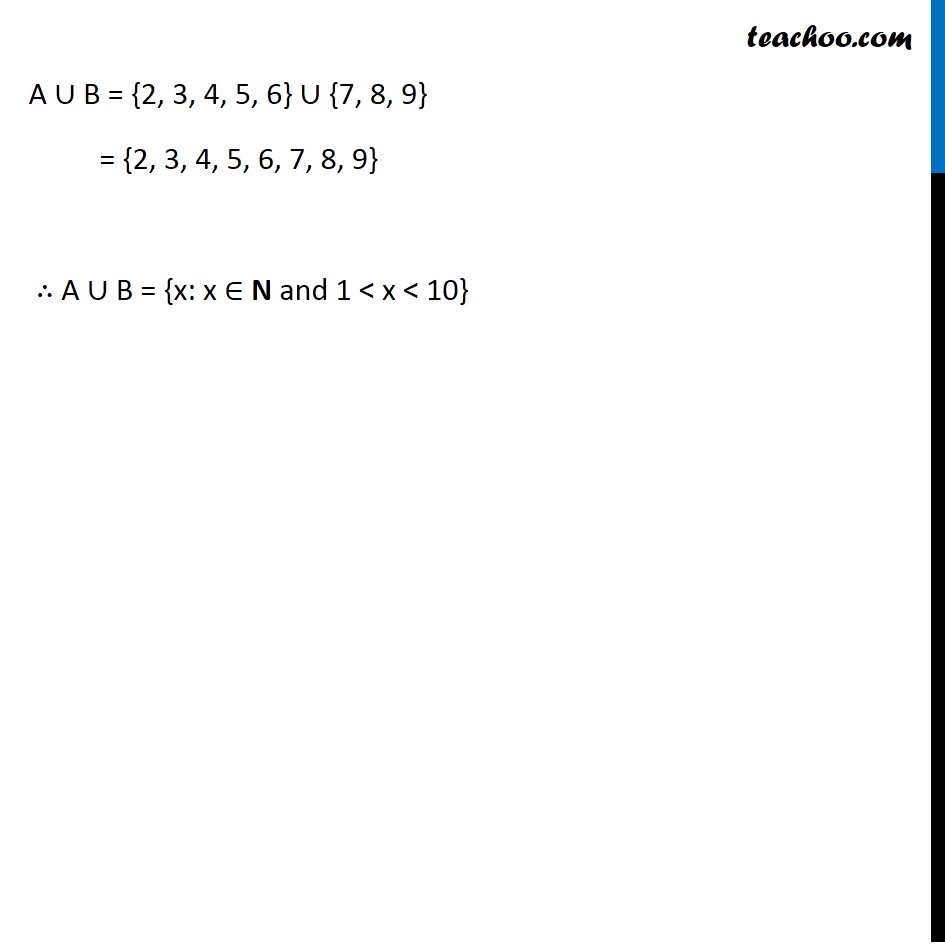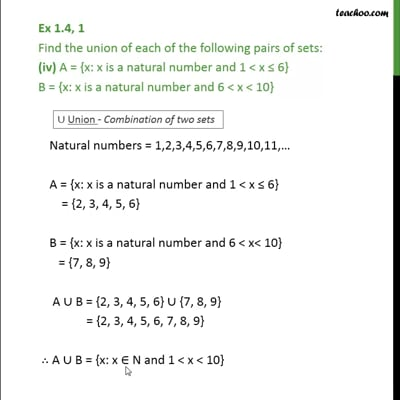Ex 1.4

Chapter 1 Class 11 Sets
Serial order wiseThis video is only available for Teachoo black users

Maths Crash Course - Live lectures + all videos + Real time Doubt solving!

### Transcript

Ex 1.4, 1 Find the union of each of the following pairs of sets: (iv) A = {x: x is a natural number and 1 < x ≤ 6} B = {x: x is a natural number and 6 < x < 10} Natural numbers = 1, 2, 3, 4, 5, 6, 7, 8, 9, 10, 11,… A = {x: x is a natural number and 1 < x ≤ 6} = {2, 3, 4, 5, 6} B = {x: x is a natural number and 6 < x< 10} = {7, 8, 9} ∪ Union - Combination of two sets A ∪ B = {2, 3, 4, 5, 6} ∪ {7, 8, 9} = {2, 3, 4, 5, 6, 7, 8, 9} ∴ A ∪ B = {x: x ∈ N and 1 < x < 10}